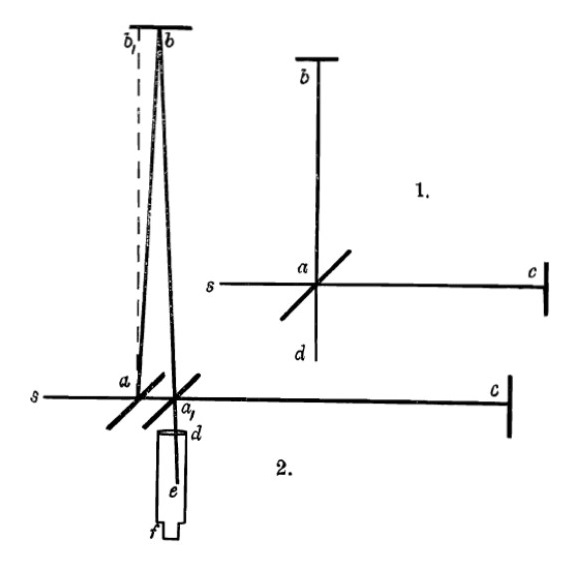# Michelson-Morley experiment

This post relates to a previous post here.

The Michelson-Morley experiment is a famous “null” result that has been understood as leading to the Lorentz transformation. However, an elementary error has persisted so that the null result is fully consistent with classical physics. Let us look at it in detail:The Michelson-Morley paper of 1887 [Amer. Jour. Sci.-Third Series, Vol. XXXIV, No. 203.–Nov., 1887] explains it using the above figures:

Let sa, fig. 1, be a ray of light which is partly reflected in ab, and partly transmitted in ac, being returned by the mirrors b and c, along ba and ca. ba is partly transmitted along ad, and ca is partly reflected along ad. If then the paths ab and ac are equal, the two rays interfere along ad. Suppose now, the ether being at rest, that the whole apparatus moves in the direction sc, with the velocity of the earth in its orbit, the directions and distances traversed by the rays will be altered thus:— The ray sa is reflected along ab, fig. 2; the angle bab, being equal to the aberration =a, is returned along ba1, (aba1 =2a), and goes to the focus of the telescope, whose direction is unaltered. The transmitted ray goes along ac, is returned along ca1, and is reflected at a1, making ca1e equal 90—a, and therefore still coinciding with the first ray. It may be remarked that the rays ba1 and ca1, do not now meet exactly in the same point a1, though the difference is of the second order; this does not affect the validity of the reasoning. Let it now be required to find the difference in the two paths aba1, and aca1.

Let V = velocity of light.
v = velocity of the earth in its orbit,
D = distance ab or ac, fig. 1.
T = time light occupies to pass from a to c.
T1 = time light occupies to return from c to a1 (fig. 2.)

The paper then goes on to give values for T and T1 incorrectly as

The correct values are as follows:

First, it is important to note that distance is the independent variable and time is the dependent variable, since the distances are fixed at the outset, such that “the paths ab and ac are equal”. Second, this entails that pace and lenticity rather than time speed and time velocity should be used, since they have distance in the denominator. This is simpler than but similar to using space speed and space velocity.

Thus instead of the addition and subtraction of velocities, one should use the addition and subtraction of lenticities. Then the correct values for T and T1 are